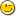### 1. unicode length

Here is a little function to report an UTF-8 string length.

```public function ulength(sequence s)
integer res
integer i, lg
atom char

i = 1
res = 0
lg = length(s)
if lg < 2 then return length(s) end if
while i <= lg do
if and_bits(s[i],#80) = #00 then
i += 1
elsif and_bits(s[i], #E0) = #C0 then
i += 2
elsif and_bits(s[i], #F0) = #E0 then
i += 3
elsif and_bits(s[i], #F8) = #F0 then
i += 4
else
i += 1
end if
res += 1
end while
return res
end function
```

It works also with ASCII strings so it could replace length().

Jean-Marc

### 2. Re: unicode length

And here is un UTF-8 compliant head() function:

```function uhead(sequence s, integer n)
sequence res
integer i, lg, ul
atom char

i = 1
ul = 0
lg = length(s)
if lg < 2 then return head(s, n) end if
while i <= lg do
if and_bits(s[i],#80) = #00 then
i += 1
elsif and_bits(s[i], #E0) = #C0 then
i += 2
elsif and_bits(s[i], #F0) = #E0 then
i += 3
elsif and_bits(s[i], #F8) = #F0 then
i += 4
else
i += 1
end if
ul += 1
if ul = n then return s[1..i-1] end if
end while
return s
end function
```

It works also with ASCII strings.

Jean-Marc

### 3. Re: unicode length

atom char is not used. (both)
sequence res is not used. (second)
integer si = s[i] might improve performance. (both)
length() needs to remain as-is for tables and other nested sequences, so "could replace length()" => "can be used instead of length() for all string-like inputs".

### 4. Re: unicode length

Thank you Pete,

With a simple mapping function, almost all UTF-8 compliant functions can be built:

```include std/types.e
include std/text.e
include std/sequence.e

constant FROM=1, UPTO=2

------------------------------------------------------------------------------

-- returns position of each utf8 character in a string as a sequence of pairs
-- {from, upto}
-- example:
-- umap("\$£€¥") = {
--   {1,1},  -- first character ranges from position 1 to position 1
--   {2,3},  -- second character ranges from position 2 to position 3
--   {4,6},  -- third character ranges from position 4 to position 6
--   {7,8}   -- fourth character ranges from position 7 to position 8
-- }

function umap(string s)
integer i = 1
sequence res = {}
while i <= length(s) do
integer si = s[i]
if and_bits(si, #80) = #00 then
res = append(res, {i, i})
i += 1
elsif and_bits(si, #E0) = #C0 then
res = append(res, {i, i+1})
i += 2
elsif and_bits(si, #F0) = #E0 then
res = append(res, {i, i+2})
i += 3
elsif and_bits(si, #F8) = #F0 then
res = append(res, {i, i+3})
i += 4
else
res = append(res, {i, i})
i += 1
end if
end while
return res
end function

------------------------------------------------------------------------------

function ulength(string s)
return length(umap(s))
end function

------------------------------------------------------------------------------

function uhead(string s, integer n)
sequence um = umap(s)
return head(s, um[n][UPTO])
end function

------------------------------------------------------------------------------

function ureverse(string s)
sequence um = umap(s)
sequence res = {}
for i = length(um) to 1 by -1 do
res &= s[um[i][FROM]..um[i][UPTO]]
end for
return res
end function

------------------------------------------------------------------------------

function uremove(string s, integer start, integer stop=start)
sequence um = umap(s)
return remove(s, um[start][FROM], um[stop][UPTO])
end function

------------------------------------------------------------------------------

function ureplace(string s, object what, integer start, integer stop=start)
sequence um = umap(s)
sequence res = remove(s, um[start][FROM], um[stop][UPTO])
return insert(res, what, um[start][FROM])
end function

------------------------------------------------------------------------------

```

This is just a subset of what can be done.

Of course, UTF-8 strings must be written with an UTF-8 encoding, so this is mostly useful on Linux. Even US programmers can need it to draw box frames.

Here is an example that compares ASCII and UTF-8 functions:

```sequence currencies = "\$£€¥"  -- dollar, pound, euro, yen
printf(1, "currencies = %s\n", {currencies})
printf(1, "umap(currencies) = %s\n", {sprint(umap(currencies))})
puts(1, "\n")
printf(1, "length(currencies) = %d\n", {length(currencies)})
printf(1, "ulength(currencies) = %d\n", {ulength(currencies)})
puts(1, "\n")
printf(1, "head(currencies, 3) = %s\n", {head(currencies, 3)})
printf(1, "uhead(currencies, 3) = %s\n", {uhead(currencies, 3)})
puts(1, "\n")
printf(1, "reverse(currencies) = %s\n", {reverse(currencies)})
printf(1, "ureverse(currencies) = %s\n", {ureverse(currencies)})
puts(1, "\n")
printf(1, "remove(currencies, 2) = %s\n", {remove(currencies, 2)})
printf(1, "uremove(currencies, 2) = %s\n", {uremove(currencies, 2)})
puts(1, "\n")
printf(1, "replace(currencies, 'E', 3) = %s\n", {replace(currencies, 'E', 3)})
printf(1, "ureplace(currencies, 'E', 3) = %s\n", {ureplace(currencies, 'E', 3)})
```

Jean-Marc

### 5. Re: unicode length

Take a look at the Euphoria Unicode branch of the SCM. Also, ulength would give the wrong result for things like a sequence of bus numbers, or temperatures. For utf32, the generic sequence functions should work out of the box, which is probably why Derek was/is an advocate for using this encoding for actually working with strings. You can prefix them or not and put them all together in one file called utf8.e .

```include utf8.e as u8

? u8:ulength("Hello")
```

I think it would probably drive me crazy if it were named length() though. ;)

### 6. Re: unicode length

SDPringle said...

which is probably why Derek was/is an advocate for using this encoding for actually working with strings.

Me too. Since UTF-32 actually only uses 31 bits, it fits perfectly with the old 31-bit integer used by 32-bit OE. (64-bit OE now uses a 63-bit integer, but even so...)

My recollection was that even C libraries like GTK would convert to UTF-32 to carry out various oeprations like checking the character length, and then only convert to UTF-8 as the final step.

### 7. Re: unicode length

jimcbrown said...

UTF-32 actually only uses 31 bits

<mutter>21 bits</mutter>Not signed in.